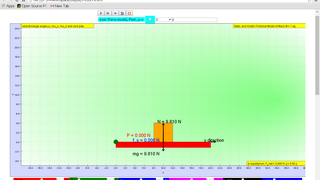Intro Page

### Translations

Code Language Translator Run### Software Requirements

SoftwareRequirements

 Android iOS Windows MacOS with best with Chrome Chrome Chrome Chrome support full-screen? Yes. Chrome/Opera No. Firefox/ Samsung Internet Not yet Yes Yes cannot work on some mobile browser that don't understand JavaScript such as..... cannot work on Internet Explorer 9 and below

### CreditsThis email address is being protected from spambots. You need JavaScript enabled to view it.; Francisco Esquembre

### end faq

http://iwant2study.org/lookangejss/02_newtonianmechanics_3dynamics/ejss_model_friction/friction_Simulation.xhtml

### Learning Outcomes

1. To explain and understand phenomena when there is no net force acting on a stationary object, or on an object that is moving in a straight line using modelling technique [diagrammatic models, mathematical models (dynamics equations), or any set of predictive and explanatory rules/principles].
2. To predict changes in motion (if any) of an object based on the forces acting on it using modelling technique [diagrammatic models, mathematical models (dynamics equations), or any set of predictive and explanatory rules/principles].

### Key inquiry question: What are the characteristics and effects of forces in nature?

1. & 2. Types of force and its effects • Friction always opposes the motion between the two surfaces in contact. The frictional force between two surfaces on a horizontal plane depends on the material in contact; surfaces in contact; force pressing the two surfaces together; independent of area of contact.

### Students’ prior knowledge of Forces

#### Primary level:

Students learn that a force:

• may be a push or a pull that acts on an object, e.g. magnetic force and gravitational force (forces that act at a distance), elastic spring force and frictional force (only when objects are in contact).

• can move a stationary object; speed up, slow down, change the direction or stop a moving object; and change the shape of objects.

Students conduct simple experiments to investigate the effects of friction on the motion of objects and forces on springs (mass on spring system), and learn how friction can be useful and can also be a problem.

#### Lower secondary level:

Students learn that:

• a force (push or pull) is exerted when one thing interacts with another, e.g. gravitational force, frictional force and magnetic force.

• forces affect the state of rest or motion and the size and shape of a body (unit: newton) and the type of force will have different effects on an object.

### Students’ common misconceptions and learning difficulties in Forces

#### Forces between masses, charges and magnets:

Students often think that:

• only active agents (usually living things) exert forces and cause motion, whereas passive forces by inert objects (e.g. a table) do not exist;

• an object moves in the direction of the strongest force rather than the direction of the resultant force; and force is a property of an object and gets used up.

#### Equilibrium of forces:

Students often think that:

• at equilibrium, forces cease to act on the object; and

• the tension in a string is the sum of the forces acting on each end.

### Video

Frictional force modelling with simulation activity by lookang lawrence wee

Imagine you put a playing card on top of a cup, then a coin on top of the playing card. Quickly pull the playing card away. What will happen to the coin? Explain your answer. Watch the video at http://tinyurl.com/ast2016-1 and check your prediction

Newtons 1st Law-coin and glass by Nick Alsleben

### Engage for Newton's 1st Law

Imagine placing a strip of tape on the floor. Then you ask a friend to run towards you from about 30 meters as fast as possible and to stop exactly on the tape. What will happen to your friend when he or she tries to stop? Explain your answer

What you demonstrated in your two thought experiments, is Newton’s First Law. It states that

“an object will remain at rest or in uniform motion in a straight line unless acted upon by an external force.”

In case 1 (video above), the coin stayed in place, as the card was pulled away so fast, that friction did not act. In case 2 (engage for newton's 1st law), your friend had some forward motion, that could not be removed immediately.

### Pre Modeling Activity

In this activity, you will be asked to draw forces in diagrams. Forces are usually indicated by arrows. The length of the arrow tells us how strong a force is: the longer the arrow, the larger the force.

In the example, we see two vertical forces acting on a Wooden Block resting on a slope. Its Fgrav= Weight is pulling it down and Fnorm = contact force on block by slope, an upward force. The Ffrict is the frictional force arising when there is a Fapp = Push to the right.  The fact that the arrows are equally large, tells us that the two forces have the same magnitude.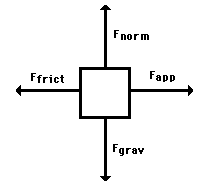### Modeling Activity

Modeling Activity using this #html5 simulation

### No Net Force (no Push Force )ModelTom has just been promoted and is pushing a file cabinet down the hall to his new office. He begins by looking at the file cabinet and considers how to best go about his task. Select Fx = 0 to model the zero force model. Note the motion of the block is no change in position and velocity is zero all the time.

### No Net Force ( 0<|Push| => Maximum Static Friction) ModelHe then begins pushing on the file cabinet, which, at first, does not move at all. To simulate this case, select Push to be 3 N and observe what happens to the motion of the block. Note that the Push is cancel out by the Friction Force. Even at Push = 4.905 N is balanced out by Friction Force and the block does not move.### Just Enough Push force (Push> Maximum Static Friction) to move Model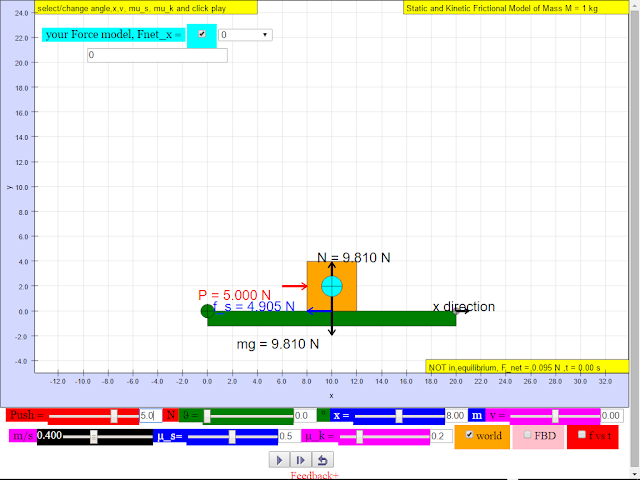He pushes it slightly harder than the maximum static friction, and it is sliding . Thus, applying a Push force just larger than 4.905 N, the object begins to slide. But at t >0, the Friction becomes Kinetic Friction.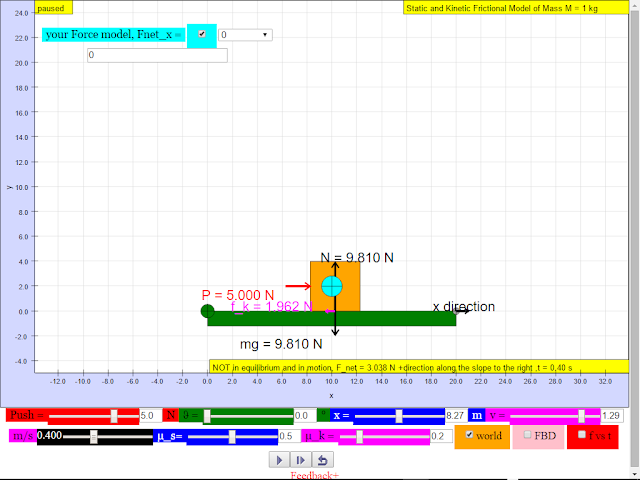Eventually the file cabinet begins to slide across the floor, slowly moving towards his new office with acceleration.### Big Idea

Thus, the evidences and model building process above suggests the condition of acceleration is the presence of non zero net force, which is F = ma, Newton's Second Law.

### Misconceptions

Students may think that it is possible to experience is pushing the file cabinet, and it is moving to the right with constant velocity.

as suggested by workshop participant from JJC, moments are calculated and reflected in simulation!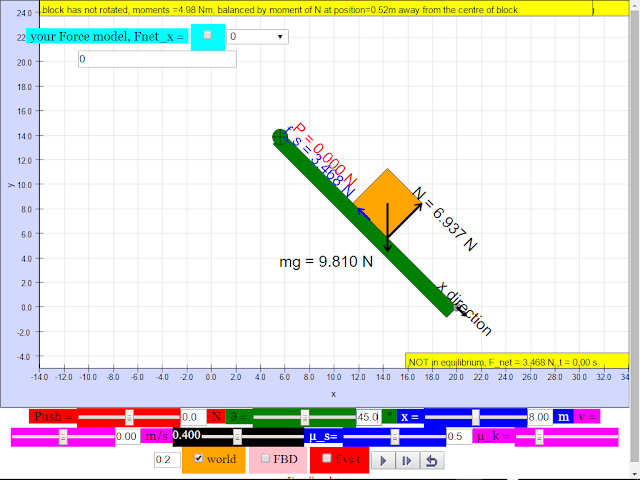angle = 45, block does not rotate, normal contact force shifts to the extreme lower end of the block, just in position to not rotate clockwise.https://iwant2study.org/ospsgx/index.php/interactive-resources/physics/02-newtonian-mechanics/02-dynamics/42-frictionangle > 45, block  rotates (but not simulated), normal contact force shifts beyond the extreme lower end of the block, in real life, block will rotate clockwise.https://iwant2study.org/ospsgx/index.php/interactive-resources/physics/02-newtonian-mechanics/02-dynamics/42-friction

### Versions

1. http://weelookang.blogspot.sg/2016/02/frictional-block-free-body-diagram-with.html BlogPost by lookang with added moments calculations.
2. http://weelookang.blogspot.com/2014/04/ejss-static-and-kinetic-friction-on.html BlogPost by lookang
3. Sliding Down an Incline Plane Model by Francisco Esquembre http://www.compadre.org/osp/items/detail.cfm?ID=9973

### Other Resources

1. http://phet.colorado.edu/en/simulation/legacy/forces-and-motion Forces and Motion by PHET
2. http://physics.bu.edu/~duffy/HTML5/static_friction.html Exploring static friction by Andrew Duffy
3. http://ophysics.com/f1.html by This email address is being protected from spambots. You need JavaScript enabled to view it.
4. http://ophysics.com/f2.html by This email address is being protected from spambots. You need JavaScript enabled to view it.